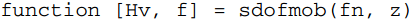### Create an Account

Home / Questions / Calculate the undamped resonance frequency fn and relative damping ζ of a mechanical sing...

# Calculate the undamped resonance frequency fn and relative damping ζ of a mechanical single-degree-of-freedom system with the following

Calculate the undamped resonance frequency fn and relative damping ζ of a mechanical single-degree-of-freedom system with the following parameters: m = 4 kg, k = 106 m/N, and c = 80 m/Ns. Use the sdofacc function from Problem 5.5 to compute the accelerance and plot it. Then use a suitable method from Section 5.5 to find the mass, stiffness and damping values. How correct can you get it? (The accuracy of particularly the relative damping estimate will be poor from a visual inspection, so do not expect a very high accuracy for c. m and k should be much easier to obtain good results for.) Hint: Use the asymptotic behavior at higher frequencies to obtain the mass, then the peak in the imaginary part to find the resonance frequency, from which the stiffness can be calculated using the mass estimate. Finally find the damping ζ using the 3-dB bandwidth and use the appropriate equation from Section 5.5 to find the viscous damping.

Problem 5.5

Write a MATLAB/Octave function with a definitionwhich calculates the mobility frequency response Hv(f) in Equation, given the undamped resonance frequency fn = ωn/2π and the relative damping ζ . Use the function to plot the mobility for fn = 1 Hz, and ζ = 0.01, 0.05, and 0.1. Plot the three frequency responses overlaid, with linear scales as well as logarithmic y-scale (using the MATLAB/Octave semilogy command), and log–log scale (log log command). Observe that with a nominal resonance frequency of 1 Hz the frequency axis will be equal to the normalized frequency r = f/fn. Then write a new MATLAB/Octave function sdofacc similar to the previous function, but which calculates the acce lerance frequency response. Plot the accelerances for the same values of frequency and damping as for mobility. Compare the results with those for mobility.

May 20 2020 View more View LessSubscribe To Get Solution